## Friday, August 10, 2007

### Irodov Problem 1.73Letbe the tension in string connected to mass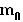and let T be the tension in the string connected to masses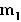and. Further letbe the acceleration of the massand let w be the acceleration of the massrelative to the moving pulley B (holding massesand) directed towards pulley B. The net acceleration of the massesandwith respect to a stationary frame areandrespectively.

Forces on mass: Theres only one force on this mass the tensionin the string over pulley A. Thus, the dynamics of the mass are given as,Forces on mass: There are two forces acting on this mass, i) the tension in the string T pulling it up and the force of gravitypulling it downwards. The net acceleration of this mass istowards the floor. Thus,Forces on mass: There are two forces acting on this mass, i) the tension in the string T pulling it up and the force of gravitypulling it downwards. The net acceleration of this mass isis towards the floor. Thus,Forces on the Pulley B:There are three forces acting on pulley B, i) tensionpulling it towards pulley A and ii) tensions T on each of the two parts of the string. The mass of the pulley is 0 and its acceleration isacting downwards. Thus, we have,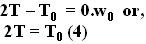Now we have all the information to solve for all the unknowns as follows,

From (1), (4) we have,From (2) and (5) we have,Similarly, from (3) and (5) we have,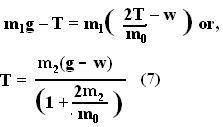From (6) and (7) we can now find w as,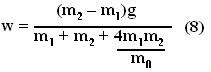Now we can use (6) and (8) to find T as,From (9) and (5) we have,Finally we can determine the accelerations of both the massesandrespectively as,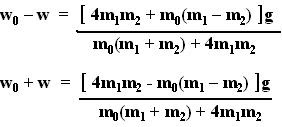Anonymous said...

thank you for the sollutionrudraneil said...

thank you for the sollutionAnonymous said...

thanks sir